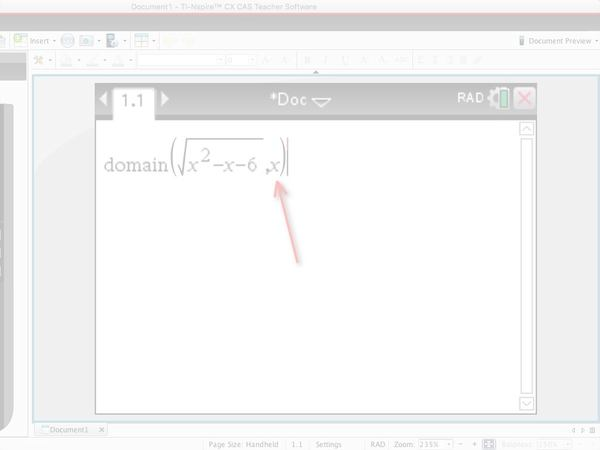# Finding the Maximal Domain on the TI-Nspire Calculator

## What is a Maximal Domain?

Domains and Ranges are a huge part of the Math Methods course. I am constantly drawing graphs to gain meaning from the problem at hand. The functions we work with in the Methods course invariably have a restricted domain. The domain may either be restricted within the question (i.e. the restricted domain is given to you) or the domain may simply have a domain that might not be R (i.e. x does not exist for some sections of the graph).

The second type of functions has what we call a maximal domain. That is, the maximum set of value for which the function exists.

• Sometimes the maximal domain is R (e.g. for y=x²).
• Sometimes the maximal domain is R+ (e.g. for y=log(x))
• Sometimes the maximal domain is restricted to a set of values [0,∞) (e.g. the domain for which y=x² has an inverse)

## When do you need to find the maximal domain?

Sometimes a skills question in Math Methods Exam 2 will simply ask you to state the maximal domain.  Some examples of these types of questions as found in VCAA papers are in the video below. Other times you will need to know what the maximal domain is in order to find the domain of the inverse or the domain of a composite function. You ALWAYS need to state the domain if a question asks for the ‘function’. E.g. “Find f’, the derivative function of f.”

## How to find the Maximal Domain on your calculator?

A maximal domain can be also found using the TI-Nspire Calculator. In the following presentation, I will walk through how you can go about using the calculator to find the maximal domain of a function.

Watch the video, then try the V.C.A.A. question found on the last slide.## How to find the Maximal Domain by hand?

Finding the maximal domain by hand is best done by drawing a graph and/or considering any places where x can not exist.

Common things to check include:

• Any expression under a square root sign – this cannot be negative
• Any expression on the denominator of a fraction – this cannot equal zero
• Any expression in a logarithmic statement – this cannot be negative e.g. ln(x) where x=-8 does not exist

If you have any questions about finding maximal domains, feel free to pop them in the comments below.

Freda Armstrong

Other V.C.A.A. Questions testing this skill can be found on their website: http://www.vcaa.vic.edu.au/Pages/vce/studies/mathematics/cas/casexams.aspx